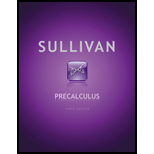# Precalculus9th Edition, Michael SullivanBuy this textbookBuy

## FREE Answers for Precalculus

### Book Details

Table of Contents 1. Graphs 1.1 The Distance and Midpoint Formulas 1.2 Graphs of Equations in Two Variables: Intercepts; Symmetry 1.3 Lines 1.4 Circles Chapter Review Chapter Test Chapter Projects 2. Functions and Their Graphs 2.1 Functions 2.2 The Graph of a Function 2.3 Properties of Functions 2.4 Library of Functions; Piecewise-defined Functions 2.5 Graphing Techniques: Transformations 2.6 Mathematical Models: Building Functions Chapter Review Chapter Test Cumulative Review Chapter Projects 3. Linear and Quadratic Functions 3.1 Linear Functions and Their Properties 3.2 Linear Models: Building Linear Functions from Data 3.3 Quadratic Functions and Their Properties 3.4 Build Quadratic models from Verbal Descriptions and from Data 3.5 Inequalities Involving Quadratic Functions Chapter Review Chapter Test Cumulative Review Chapter Projects 4. Polynomial and Rational Functions 4.1 Polynomial Functions and Models 4.2 Properties of Rational Functions 4.3 The Graph of a Rational Function 4.4 Polynomial and Rational Inequalities 4.5 The Real Zeros of a Polynomial Function 4.6 Complex Zeros: Fundamental Theorem of Algebra Chapter Review Chapter Test Cumulative Review Chapter Projects 5. Exponential and Logarithmic Functions 5.1 Composite Functions 5.2 One-to-One Functions; Inverse Functions 5.3 Exponential Functions 5.4 Logarithmic Functions 5.5 Properties of Logarithms 5.6 Logarithmic and Exponential Equations 5.7 Financial Models 5.8 Exponential Growth and Decay Models; Newton's Law; Logistic Growth and Decay Models 5.9 Building Exponential, Logarithmic, and Logistic Functions from Data Chapter Review Chapter Test Cumulative Review Chapter Projects 6. Trigonometric Functions 6.1 Angles and Their Measure 6.2 Trigonometric Functions: Unit Circle Approach 6.3 Properties of the Trigonometric Functions 6.4 Graphs of the Sine and Cosine Functions 6.5 Graphs of the Tangent, Cotangent, Cosecant, and Secant Functions 6.6 Phase Shift; Sinusoidal Curve Fitting Chapter Review Chapter Test Cumulative Review Chapter Projects 7. Analytic Trigonometry 7.1 The Inverse Sine, Cosine, and Tangent Functions 7.2 The Inverse Trigonometric Functions (continued) 7.3 Trigonometric Equations 7.4 Trigonometric Identities 7.5 Sum and Difference Formulas 7.6 Double-angle and Half-angle Formulas 7.7 Product-to-Sum and Sum-to-Product Formulas Chapter Review Chapter Test Cumulative Review Chapter Projects 8. Applications of Trigonometric Functions 8.1 Applications Involving Right Triangles 8.2 Law of Sines 8.3 Law of Cosines 8.4 Area of a Triangle 8.5 Simple Harmonic Motion; Damped Motion; Combining Waves Chapter Review Chapter Test Cumulative Review Chapter Projects 9. Polar Coordinates; Vectors 9.1 Polar Coordinates 9.2 Polar Equations and Graphs 9.3 The Complex Plane; DeMoivre's Theorem 9.4 Vectors 9.5 The Dot Product 9.6 Vectors in Space 9.7 The Cross Product Chapter Review Chapter Test Cumulative Review Chapter Projects 10. Analytic Geometry 10.1 Conics 10.2 The Parabola 10.3 The Ellipse 10.4 The Hyperbola 10.5 Rotation of Axes; General Form of a Conic 10.6 Polar Equations of Conics 10.7 Plane Curves and Parametric Equations Chapter Review Chapter Test Cumulative Review Chapter Projects 11. Systems of Equations and Inequalities 11.1 Systems of Linear Equations: Substitution and Elimination 11.2 Systems of Linear Equations: Matrices 11.3 Systems of Linear Equations: Determinants 11.4 Matrix Algebra 11.5 Partial Fraction Decomposition 11.6 Systems of Nonlinear Equations 11.7 Systems of Inequalities 11.8 Linear Programming Chapter Review Chapter Test Cumulative Review Chapter Projects 12. Sequences; Induction; The Binomial Theorem 12.1 Sequences 12.2 Arithmetic Sequences 12.3 Geometric Sequences; Geometric Series 12.4 Mathematical Induction 12.5 The Binomial Theorem Chapter Review Chapter Test Cumulative Review Chapter Projects 13. Counting and Probability 13.1 Counting 13.2 Permutations and Combinations 13.3 Probability Chapter Review Chapter Test Cumulative Review Chapter Projects 14. A Preview of Calculus: The Limit, Derivative, and Integral of a Function 14.1 Finding Limits Using Tables and Graphs 14.2 Algebra Techniques for Finding Limits 14.3 One-sided Limits; Continuous Functions 14.4 The Tangent Problem; The Derivative 14.5 The Area Problem; The Integral Chapter Review Chapter Test Chapter Projects Appendix A. Review A.1 Algebra Essentials A.2 Geometry Essentials A.3 Polynomials A.4 Synthetic Division A.5 Rational Expressions A.6 Solving Equations A.7 Complex Numbers; Quadratic Equations in the Complex Number System A.8 Problem Solving: Interest, Mixture, Uniform Motion, Constant Rate Job Applications A.9 Interval Notation; Solving Inequalities A.10 nth Roots; Rational Exponents Appendix B. Graphing Utilities B.1 The Viewing Rectangle B.2 Using a Graphing Utility to Graph Equations B.3 Using a Graphing Utility to Locate Intercepts and Check for Symmetry B.4 Using a Graphing Utility to Solve Equations B.5 Square Screens B.6 Using a Graphing Utility to Graph Inequalities B.7 Using a Graphing Utility to Solve Systems of Linear Equations B.8 Using a Graphing Utility to Graph a Polar Equation B.9 Using a Graphing Utility to Graph Parametric Equations

## More Editions of This Book

Corresponding editions of this textbook are also available below:
PRECALC. MYMATHLAB NVCC ACCESS CODE
1 Edition
ISBN: 9780135351499
EBK PRECALCULUS
11 Edition
ISBN: 9780135228982
Precalculus
11 Edition
ISBN: 9780135189405
Mylab Math With Pearson Etext -- Standalone Access Card -- For Precalculus (11th Edition)
11 Edition
ISBN: 9780135189795
PRECALCULUS(LL)W/18 WK.ACCESS
11 Edition
ISBN: 9780136167716
Precalculus (10th Edition)
10 Edition
ISBN: 9780321979070
Precalculus Plus MyLab Math with eText -- Access Card Package (10th Edition) (Sullivan & Sullivan Precalculus Titles)
10 Edition
ISBN: 9780321978981
Precalculus, 7th Edition
7 Edition
ISBN: 9780131517448
Precalculus
8 Edition
ISBN: 9780132256889
Precalculus
5 Edition
ISBN: 9780130231413
Precalculus
4 Edition
ISBN: 9780138407452
Precalculus
1 Edition
ISBN: 9780536612397
Precalculus, Second Custom Edition For George Mason University
2 Edition
ISBN: 9781323137963© 2021 bartleby. All Rights Reserved.